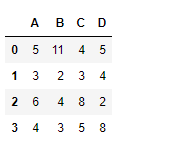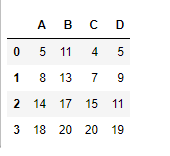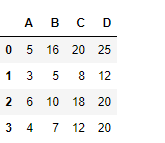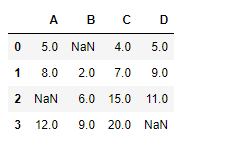# Python | Pandas dataframe.cumsum()

• Last Updated : 16 Nov, 2018

Python is a great language for doing data analysis, primarily because of the fantastic ecosystem of data-centric python packages. Pandas is one of those packages and makes importing and analyzing data much easier.

Pandas` dataframe.cumsum()` is used to find the cumulative sum value over any axis. Each cell is populated with the cumulative sum of the values seen so far.

Syntax: DataFrame.cumsum(axis=None, skipna=True, *args, **kwargs)

Parameters:
axis : {index (0), columns (1)}
skipna : Exclude NA/null values. If an entire row/column is NA, the result will be NA

Returns: cumsum : Series

Example #1: Use `cumsum()` function to find the cumulative sum of the values along the index axis.

 `# importing pandas as pd ` `import` `pandas as pd ` ` `  `# Creating the dataframe ` `df ``=` `pd.DataFrame({``"A"``:[``5``, ``3``, ``6``, ``4``], ` `                   ``"B"``:[``11``, ``2``, ``4``, ``3``], ` `                   ``"C"``:[``4``, ``3``, ``8``, ``5``], ` `                   ``"D"``:[``5``, ``4``, ``2``, ``8``]}) ` ` `  `# Print the dataframe ` `df `

Output :Now find the cumulative sum of the values over the index axis

 `# To find the cumulative sum ` `df.cumsum(axis ``=` `0``) `

Output :Example #2: Use `cumsum()` function to find the cumulative sum of the values seen so far along the column axis.

 `# importing pandas as pd ` `import` `pandas as pd ` ` `  `# Creating the dataframe ` `df ``=` `pd.DataFrame({``"A"``:[``5``, ``3``, ``6``, ``4``], ` `                   ``"B"``:[``11``, ``2``, ``4``, ``3``], ` `                   ``"C"``:[``4``, ``3``, ``8``, ``5``], ` `                   ``"D"``:[``5``, ``4``, ``2``, ``8``]}) ` ` `  `# To find the cumulative sum along column axis ` `df.cumsum(axis ``=` `1``) `

Output :Example #3: Use `cumsum()` function to find the cumulative sum of the values seen so far along the index axis in a data frame with `NaN` value present in dataframe.

 `# importing pandas as pd ` `import` `pandas as pd ` ` `  `# Creating the dataframe ` `df ``=` `pd.DataFrame({``"A"``:[``5``, ``3``, ``None``, ``4``], ` `                   ``"B"``:[``None``, ``2``, ``4``, ``3``], ` `                   ``"C"``:[``4``, ``3``, ``8``, ``5``], ` `                   ``"D"``:[``5``, ``4``, ``2``, ``None``]}) ` ` `  `# To find the cumulative sum ` `df.cumsum(axis ``=` `0``, skipna ``=` `True``) `

Output :The output is a dataframe with cells containing the cumulative sum of the values seen so far along the index axis. Any `Nan` value in the dataframe is skipped.

My Personal Notes arrow_drop_up
Recommended Articles
Page :